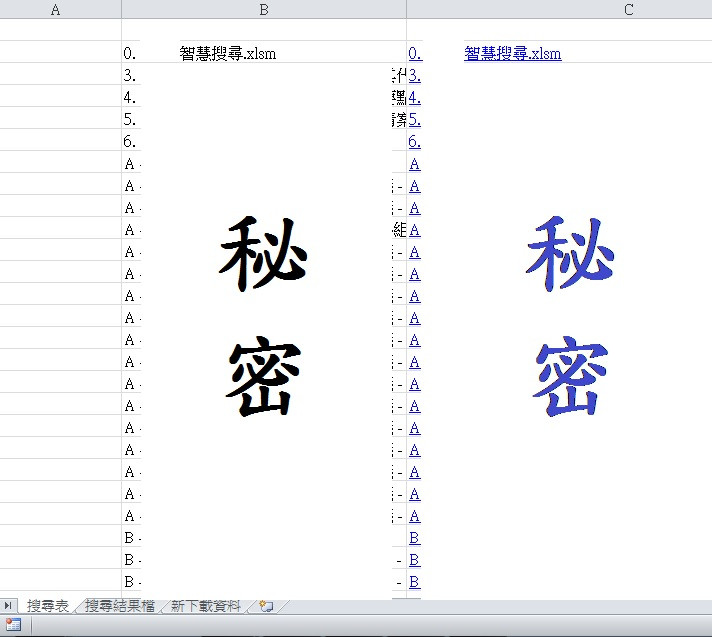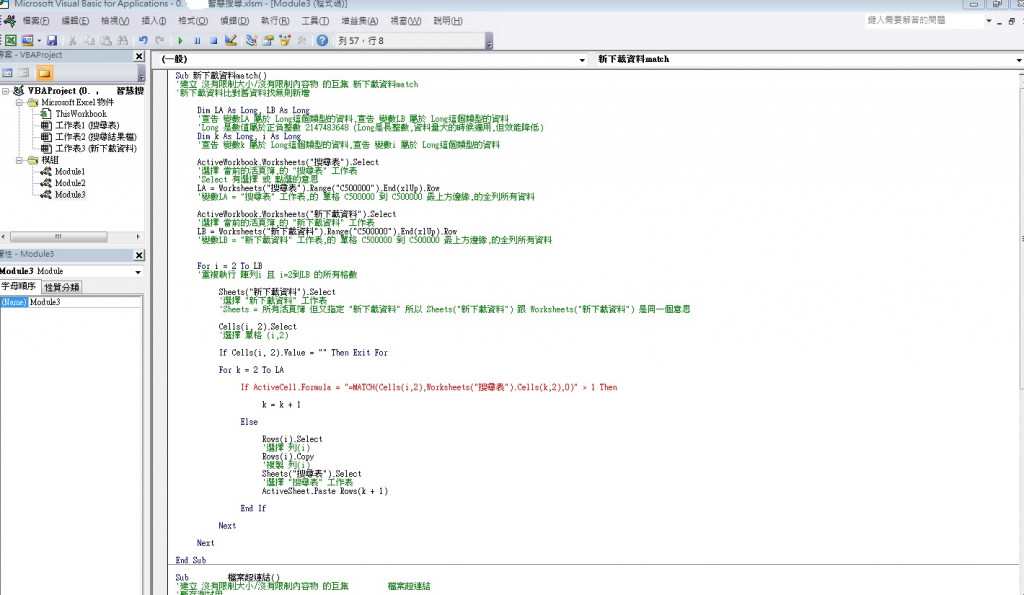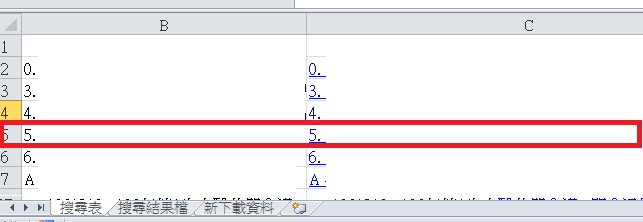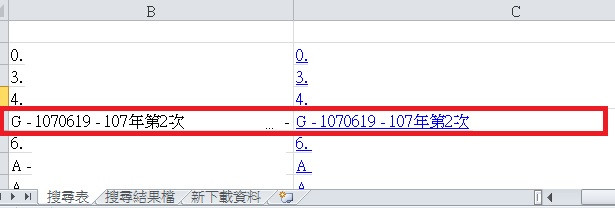#0

## 兩個不同的工作表比對和新增

Sub 新下載資料match()

`````` Dim LA As Long, LB As Long
Dim k As Long, i As Long

ActiveWorkbook.Worksheets("搜尋表").Select
LA = Worksheets("搜尋表").Range("C500000").End(xlUp).Row

ActiveWorkbook.Worksheets("新下載資料").Select
LB = Worksheets("新下載資料").Range("C500000").End(xlUp).Row

For i = 2 To LB
Sheets("新下載資料").Select
Cells(i, 2).Select

If Cells(i,2).Value = "" Then Exit For

For k = 2 To LA
If ActiveCell.Formula = "=MATCH(Cells(i,2),Worksheets("搜尋表").Cells(k,2),0)" > 1 Then
k=k+1
Else
Rows(i).Select
Rows(i).Copy
Sheets("搜尋表").Select
ActiveSheet.Paste Rows(k+1)
End If
Next
Next

以上 ,感恩 !!! ^^
``````

art4444 iT邦新手 5 級 ‧ 2018-09-07 10:49:38 檢舉

art4444 iT邦新手 5 級 ‧ 2018-09-07 10:49:39 檢舉

### 1 個回答

0

``````If ActiveCell.Formula = "=MATCH(Cells(i,2),Worksheets("搜尋表").Cells(k,2),0)" > 1 Then
``````

``````Sub 新下載資料match()

Dim LA As Long, LB As Long, old as range
Dim k As Long, i As Long

LA = Worksheets("搜尋表").cells(rows.count, 2).End(xlUp).Row
set old = Worksheets("搜尋表").range(Worksheets("搜尋表").range("B2"), Worksheets("搜尋表").cells(LA, 2))
LB = Worksheets("新下載資料").cells(rows.count, 2).End(xlUp).Row

For i = 2 To LB
With Worksheets("新下載資料").Cells(i, 2)

If .Value = "" Then
Exit For
Elseif iserror(application.match(.value, old ,0)) Then
Worksheets("搜尋表").cells(rows.count, 2).end(xlup).offset(1).value = .value
Else
End If
End with
Next i
End Sub
``````

art4444 iT邦新手 5 級 ‧ 2018-08-15 16:07:18 檢舉

``````If cells(i,2).value = Worksheets("搜尋表").cells(k,2).value Then
``````
art4444 iT邦新手 5 級 ‧ 2018-08-16 11:21:47 檢舉art4444 iT邦新手 5 級 ‧ 2018-08-16 11:26:51 檢舉

art4444 iT邦新手 5 級 ‧ 2018-08-16 11:33:22 檢舉

art4444 iT邦新手 5 級 ‧ 2018-08-16 12:00:24 檢舉

Sub 新下載資料match()

`````` Dim LA As Long, LB As Long
Dim k As Long, i As Long
ActiveWorkbook.Worksheets("搜尋表").Select
LA = Worksheets_
("搜尋表").Range("C500000").End(xlUp).Row

ActiveWorkbook.Worksheets("新下載資料").Select
LB = Worksheets_
("新下載資料").Range("C500000").End(xlUp).Row

For i = 2 To LB

Sheets("新下載資料").Select
Cells(i, 2).Select

If Cells(i,2).Value = "" Then Exit For

For k = 2 To LA

If Worksheets("新下載資料").cells(i,2).value = Worksheets("搜尋表").cells(k,2).value Then Exit For

ElseIf Cells(k,2).Value = "" Then

Rows(i).Select
Rows(i).Copy
Sheets("搜尋表").Select
ActiveSheet.Paste Rows(k)

End If

Next

Next
``````

End Sub

art4444 iT邦新手 5 級 ‧ 2018-08-16 15:58:10 檢舉

art4444 iT邦新手 5 級 ‧ 2018-08-16 16:11:35 檢舉art4444 iT邦新手 5 級 ‧ 2018-08-16 16:16:01 檢舉

"新下載資料" 的3筆資料, 分別在第2.3.4列.

"搜尋表" 的281筆資料, 分別在第2.3.4...282列.

art4444 iT邦新手 5 級 ‧ 2018-09-03 16:37:12 檢舉

art4444 iT邦新手 5 級 ‧ 2018-09-07 11:07:58 檢舉

iferror這裡會出錯~

art4444 iT邦新手 5 級 ‧ 2018-09-07 14:29:32 檢舉

my typo `iserror`

art4444 iT邦新手 5 級 ‧ 2018-09-10 13:50:14 檢舉